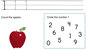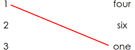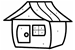# Learning Numbers

## Numbers worksheets from one to 20

Print out these free pdf worksheets to help your kids learn to recognize, read and write numbers from 1-20.  These worksheets prepare students for learning to count and perform simple math.

## Learn the numbers from one to tenEach of these numbers worksheets introduces a specific number.  Ten worksheets, one each for the numbers 1 to 10.where

## Print the numbers (1-20)20 number printing worksheets where students can practice tracing and printing numbers (as numbers and number words). One worksheet for each number 1-20.

## Match numbers to their wordsIn these number words worksheets students match numbers to their corresponding number words.  2 worksheets for the numbers 1-10, and 2 worksheets for the numbers 1-20.

## Color by numberSome color by number worksheets where students color a picture according to the numbers and directions.  A fun way to improve number recognition.

## More numbers worksheets

Explore all of our learning numbers worksheets (recognizing and printing numbers), counting worksheets (counting objects, skip counting, counting backwards) and comparing numbers worksheets ("more than", "less than", ordering numbers).

## What is K5?

K5 Learning offers reading and math worksheets, workbooks and an online reading and math program for kids in kindergarten to grade 5.  We help your children build good study habits and excel in school.